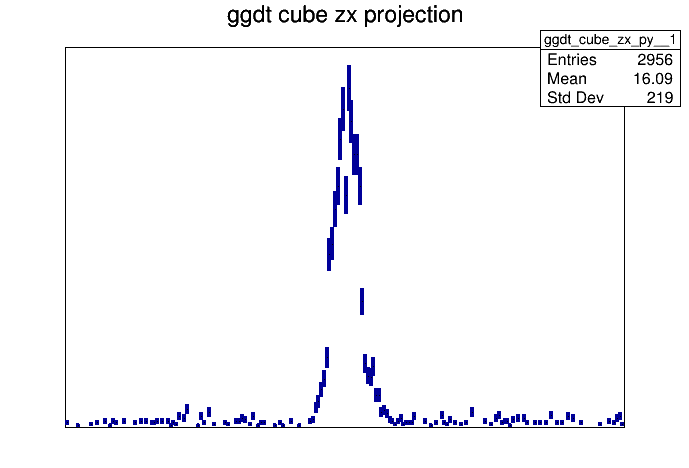# Combining two canvases into one

ROOT Version: 6.18
Platform: Not Provided
Compiler: Not Provided

Good day

I am trying to combine two canvases obtained from a cube into one canvas. So from my cube I do this:
root  ggdt_cube->GetYaxis()->SetRangeUser(386.24,406.24)
root  TH2D* matrix3 = static_cast<TH2D*>(ggdt_cube->Project3D(“zx”))
root  matrix3->Draw(“colz”)
and then I Project onto Y axis to get a 1D histogram plotted on a canvas with a default name c1.

Now I follow the same process but with a different gate : ggdt_cube->GetYaxis()->SetRangeUser(1114.24,113424)
and still get a D histogram plotted on a canvas with a default name c1.

I am trying to plot these on the same canvas but using Draw(“same”) does not work since c1 is the default name in both cases. Please help.

Hi @lum_totzin,
can you provide a minimal reproducer for your problem?

using Draw(“same”) does not work since c1 is the default name in both cases

Drawing two histograms on the same canvas is done by drawing the first with `Draw(options..)` and the second with `Draw("SAME")` – the name of the canvas they are drawn onto should be the same in both cases if you want them to appear on the same canvas. I probably misunderstand your comment.

Cheers,
Enrico

Hi Enrico

So its actually two canvases that I want to plot into one canvas otherwise what you said could have worked.

So I have a cube in which I set a gate as follows:

root  ggdt_cube->GetYaxis()->SetRangeUser(386.24,406.24)
I the get a 2D matrix after doing this:

root  TH2D* matrix3 = static_cast<TH2D*>(ggdt_cube->Project3D(“zx”))

I then project onto the matrix to get a 1D histogram. Now when I save this as a rootfile it saves as canvas e.g c1.
I then do the same procedure with a different gate i.e.

root  ggdt_cube->GetYaxis()->SetRangeUser(1114,1134)

I gate a different histogram that I want to plot together with the first one again when I save this as a rootfile it saves a canvas c1. And the challange is that I cannot plot the two into one canvas.

You can extract the actual histogram objects from the file and plot those. TCanvas::GetPrimitive should work for that purpose.

I see. Thank you very much. I will give it a try.

I am trying to use TCanvas::GetPrimitive to extract histograms from the canvas but then I get an error
*** Break *** segmentation violation

My root file is gate1124Proj396.root
The histogram title is ggdt_cube_zx_pyConvertcanv.C (221 Bytes) gate1124Proj396.C (17.1 KB)

That’s most probably because you are dereferencing a null pointer. Does `GetPrimitive` return a valid pointer?

I am not exactly sure to check if I am doing this right, since I am a beginner. But i tried the following on the code:

if ( c1==NULL ) {
std::cout << "Can not access canvas! " << c1 << std::endl ;
return NULL ;
}
c1->GetListOfPrimitives()->Print();

It returns this:
Error in : Trying to dereference null pointer or trying to call routine taking non-null arguments.
Execution of your code was aborted.
In file included from input_line_8:1:
/home/lmsebi/Documents/Cube2020/Convertcanv.C:9:4: warning: null passed to a callee that requires a non-null argument [-Wnonnull]
htwo->Draw();

If the error message points to `htwo->Draw()`, it probably means that `htwo` is a nullptr.
You should double-check that `GetPrimitive` returns what you expect (i.e. not a nullptr).

If you can share `gate1124Proj396.root` with me I can also try to retrieve that histogram from the canvas and see what the right invocation is.

Cheers,
Enrico

Oh i see. I have attached the file.

gate1124Proj396.root (9.4 KB)

Ok as you see from `c1->GetListOfPrimitives()->Print()` the name of the histogram is actually `"ggdt_cube_zx_py__1"`.

For easier access you might want to save directly the `TH1` to file rather than saving the canvas. To save a histogram to a file, in general this is sufficient:

``````TFile f("myfile.root", "recreate");
TH1F h("myhisto", "myhisto", 100, 0, 1);
h.Write();
f.Close();
``````

Hope this helps!
Enrico

This returns an empry histogram i.e. myhisto is empty and is not saved.

My simple example code writes an empty histogram, it was just to show how to write histograms to file.
As you can see the histogram is created but I don’t fill it with anything.

It should definitely be saved to file though, i.e. the file `myfile.root` should contain object `myhisto`.

P.S.
You can add some `h.Fill(0.5); h.Fill(0.3);` calls to fill the histogram with some values for testing purposes.

P.P.S.
But again, to solve your original problem, you can extract the histogram from the cavas with `GetPrimitive("ggdt_cube_zx_py__1")`

Actually this still gives an error
warning: null passed to a callee that requires a non-null argument [-Wnonnull]
htwo = (TH1F*)c1->GetPrimitive(“ggdt_cube_zx_py__1”);

We must be doing something different thenThis works, from the terminal:

`````` ~/Downloads root -l gate1124Proj396.root                                 (cern-root)
root 
Attaching file gate1124Proj396.root as _file0...
(TFile *) 0x55a94464df20
root  _file0->ls()
TFile**		gate1124Proj396.root
TFile*		gate1124Proj396.root
KEY: TCanvas	c1;1	c1
root  c1->GetListOfPrimitives()->Print()
Collection name='TList', class='TList', size=3
TFrame  X1=-1020.000000 Y1=0.000000 X2=1020.000000 Y2=250.984965 FillStyle=1001
TH1.Print Name  = ggdt_cube_zx_py__1, Entries= 2956, Total sum= 2962
TPaveText  X1=-398.578011 Y1=261.811078 X2=398.578011 Y2=280.789432
Collection name='TList', class='TList', size=1
Text  X=0.000000 Y=0.000000 Text=ggdt cube zx projection Font=0 Size=0.000000 Color=0 Align=0
root  auto h1 = (TH1F*)c1->GetPrimitive("ggdt_cube_zx_py__1")
(TH1F *) @0x7ffcefeafb98
``````

note that `h1` is not a nullptr (i.e. not zero).

I can get the mean of `h1` and also draw it in a new canvas:

``````root  h1->GetMean()
(double) 16.085271
root  TCanvas c
(TCanvas &) Name: c1 Title: c1
root  h1->Draw("A")
``````

which gets meWhat am I doing differently?

Cheers,
Enrico

1 Like

Thanks .Using

``````auto h1 = (TH1F*)c1->GetPrimitive("ggdt_cube_zx_py__1")
``````